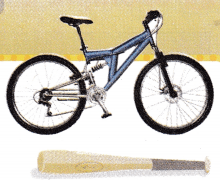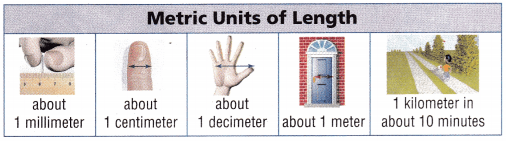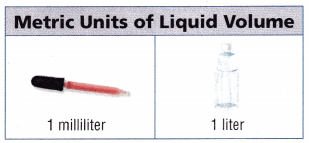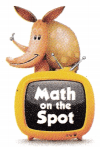# Texas Go Math Grade 4 Lesson 15.1 Answer Key Measurement Benchmarks

Refer to our Texas Go Math Grade 4 Answer Key Pdf to score good marks in the exams. Test yourself by practicing the problems from Texas Go Math Grade 4 Lesson 15.1 Answer Key Measurement Benchmarks.

## Texas Go Math Grade 4 Lesson 15.1 Answer Key Measurement Benchmarks

Essential Question

How can you use benchmarks to understand the relative sizes of measurement units?
benchmarks can be defined as the standard or reference point against which something can be measured, compared, or assessed. Benchmark numbers are numbers against which other numbers or quantities can be estimated and compared.
Benchmarks for Metric Units The metric system is based on place value. Each unit is 10 times as large as the next smaller unit. Below are some common metric benchmarks.

Unlock the ProblemA mile is a customary unit for measuring length or distance. The benchmark shows the distance you can walk in about 20 minutes.

Jake says the length of his bike is about four yards. Use the benchmark units below to determine if Jake’s statement is reasonable.A baseball bat is about one yard long. Since lake’s bike is shorter than four times the length of a baseball bat, his bike is shorter than four yards long.

So, Jake’s statement __________ reasonable.
Jake’s bike is about __________ baseball bats long.
So, Jake’s statement is not reasonable.
Jake’s bike is about 1 baseball bats long.

Example 1 Use the benchmark customary units.About how much liquid is in a mug of hot chocolate? ____________
A mug of hot chocolate is about 8 fluidounces as 1 cup can hold 8 fluid ounces.About how much does a grape fruit weigh? ___________
A grape fruit weighs about 1 ounce as it is comparatively similar to the weight of a pencil.

Math Talk

Mathematical Processes
Order the units of weight from heaviest to lightest. Use benchmarks to explain your answer.
Ton, pound and ounce. I use the benchmarks to order them a car is heavier than bread and a bread is heavier than a pencil.

Benchmarks for Metric Units The metric system is based on place value. Each unit is 10 times as large as the next smaller unit. Below are some common metric benchmarks.

Example 2 Use the benchmark metric units.A kilometer is a metric unit for measuring length or distance. The benchmark shows the distance you can walk in about 10 minutes.

Is the length of your classroom greater than or less than one kilometer?
The length of my classroom is less than 1 kilometer. As the length of the will not take 10 minutes to walk along the classroom.About how much medicine is usually in a medicine bottle?
A medicine bottle is about 120 milliliters as a medicine bottle will be small in size.About how much is the mass of a paper clip?
The mass of a paperclip is about a gram as it is comparatively similar to the weight of a currency note.

Math Talk

Mathematical Processes
Explain how benchmark measurements can help you decide which unit to use when measuring.
According the thing we have to decide which benchmark is to be used
Example
water in liquids
length in units
centimeter or meter
vegetables in gallons

Share and Show

Use benchmarks to choose the customary or the metric unit you would use to measure each.Question 1.
length of a football field
The length of a football field will be measured in the metric unit meters.

Question 2.
length of a cell phone
The length of a cell phone is measured in the customary unit inch.

Circle the better estimate.

Question 3.
width of a teacher’s desk 10 meters or 1 meter
The width of a teachers desk would be 1 meter long.

Question 4.
the amount of liquid a fish tank holds 10 cups or 10 gallons
The amount of liquid a fish tank holds is 10 gallons.

Question 5.
distance between Seattle and San Francisco 6 miles or 680 miles
The distance between Seattle and San Francisco is 680 miles.

Math Talk

Mathematical Processes
Explain why you would use kilometers instead of meters to measure the distance across the United States.
I use kilometers to measure the distance across the United States not meters because it takes so many minutes to measure the distance of United States. A kilometer is the distance that a person can travel in 10 minutes. So, it will be better if we measure the distance in kilometers if it is very long.

Problem Solving

Question 6.H.O.T. Apply Cristina is making macaroni and cheese for her family. Would Cristina use 1 pound of macaroni or 1 ounce of macaroni?
Cristina can use 1 pound of macaroni to make macaroni for her family because 1 ounce is very less and 1 pound is correct measure to cook for her family.

Question 7.
H.O.T. Write Math Dalton used benchmarks to estimate that there are more cups than quarts in one gallon. Is Dalton’s estimate reasonable? Explain.
Yes Math Dalton’s estimate is reasonable as cup is a smaller measurement than a quarts. So, there will be more cups than quarts in one gallon.

Question 8.
A mini-chameleon is about 1 inch long. ¡t spends much of its time on the ground hiding tinder leaves. Which is the best estimate for the length of a leaf the mini-chameleon might hide under?
(A) 4 inches
(B) 4 ounces
(C) 4 miles
(D) 4 pounds
(A) 4 inches

Explanation:
4 inches is the best estimate for the length of a leaf the mini-chameleon might hide under as the length of the chameleon is only 1 inch it can easily hide under the 4 inch leaf.

Question 9.
Mrs. Parker paints the school library. Which is the best estimate for the amount of paint she uses?
(A) 18 meters
(B) 18 millimeters
(C) 18 liters
(D) 18 milliliters
(C) 18 liters

Explanation:
18 liters is the best estimate for the amount of paint Mrs. Parker uses to paint the school library as it will be big and meter is not the  unit of measuring liquid and milliliters is very small unit.

Question 10.
Roger took his pet canary to the vet. Which is the best estimate of the weight of the canary?
(A) 2o pounds
(B) 20 ounces
(C) 20 feet
(D) 20 inches
(B) 20 ounces

Explanation:
The best estimate of the weight of the Roger’s pet canary is 20 ounces as pound is very big unit of measurement and feet and iches are not the units of measuring weight.

TEXAS Test Prep

Question 11.
Which is the best estimate for a dose of medicine?
(A) 2 milliliters
(B) 2 liters
(C) 2 millimeters
(D) 2 meters
(A) 2 milliliters

Explanation:
2 milliliters is the best estimate for a dose of medicine as liters is very big measurement and millimeters and meters are not the units of measuring medicine.

### Texas Go Math Grade 4 Lesson 15.1 Homework and Practice Answer Key

Circle the better estimate.

Question 1.
width of a computer mouse 2 inches or 2 feet
The width of a computer mouse is 2 inches. 2 feet is very big compared to inches.

Question 2.
weight of a bag of grapes 3 ounces or 3 pounds
Weight of a bag of grapes will be 3pounds as ounces are very small unit of measurement.

Question 3.
height of a stop sign 3 meters or 3 kilometers
The height of a stop sign is 3 meters as it will be small.

Question 4.
mass of a mouse
30 grams or 30 kilograms
The mass of a mouse is 30 grams as a mouse will be small in size.

Problem Solving

Question 5.
Which is the better estimate of the height of a basketball hoop, 10 feet or 10 yards?
The best estimate of the height of a basketball hoop 10 feet long as yard is very big compared to feet.

Question 6.
Which is the better estimate of the mass of a wrist watch, 50 grams or 50 kilograms?
The better estimate of the mass of a wrist watch is 50 grams as a watch will be small.

Question 7.
Which is the better estimate of the amount of balloon holds, 1 liter or 1 milliliter?
The better estimate of the amount of balloon holds is 1 liter as 1 milliliter is very small.

Question 8.
Which is the better estimate of the weight of a football helmet, 3 pounds or 3 ounces?
The better estimate of the weight of a football helmet is 3 pounds as ounce is a small unit of measurement when compared to pound.

Question 9.
Sandy used benchmarks to estimate that there are more inches than feet in one yard. Is Sandy’s estimate reasonable? Explain.
Yes, Sandy’s estimate is reasonable as inches is smaller unit of measurement than feet. SO, there will be more inches than feet in one yard.

Question 10.
Jonathan thinks that he rides his bicycle about 4 meters from his house to school each day. Is Jonathan’s estimate reasonable?
Explain.
Yes he is true,
Jonathan thinks that he rides his bicycle about 4 meters from his house to school each day
because distance can be measured in meters.

Question 11.
Victoria is building a box for her CDs. Which is the best estimate of the width of a CD?
(A) 5 miles
(B) 5 feet
(C) 5 yards
(D) 5 inches
Explanation:
The length of CD box will be small
so, they cannot measure in miles, feet, or yards

Question 12.
Darius is filling a jug with water to take on his trip. Which is the best estimate of the amount of water his jug holds?
(A) 4 gallons
(B) 4 cups
(C) 4 pounds
(D) 4 ounces
Explanation:
Cups is the correct choice
Because 1 cup = 8 ounces
so it will be less
pounds and gallons are not measured in liquids

Question 13.
York School has a new jungle gym and a new swing set on its playground. Which is the best estimate of the height of the jungle gym?
(A) 3 kilograms
(B) 3 meters
(C) 3 kilometers
(D) 3 grams
Explanation:
Grams and kilograms are not used in measuring
kilometers will be very long distance

Question 14.
Anna is driving from Dallas to Austin. Which is the best estimate of the distance she will drive?
(A) 320 kilograms
(B) 320 meters
(C) 320 liters
(D) 320 kilometers
Explanation:
Liters and kilograms are not measured in distance
meters will be very small
so, kilometers is correct from one place to other place to find the distance

Question 15.
Multi-Step Stan wants to know the weight of his hammer and its length. Which two units should he use?
(A) ounces and inches
(B) pounds and feet
(C) pounds and miles
(D) ounces and feet
Explanation:
1 feet = 12 inches
the hammers length will be more than 12 inches
and 1 pound = 16 ounces
the hammers weight will be more than 16 ounces

Question 16.
Multi-Step Jodi wants to weigh her dog and measure its height. Which two units should she use?
(A) pounds and inches
(B) ounces and yards
(C) kilograms and kilometers
(D) grams and millimeters